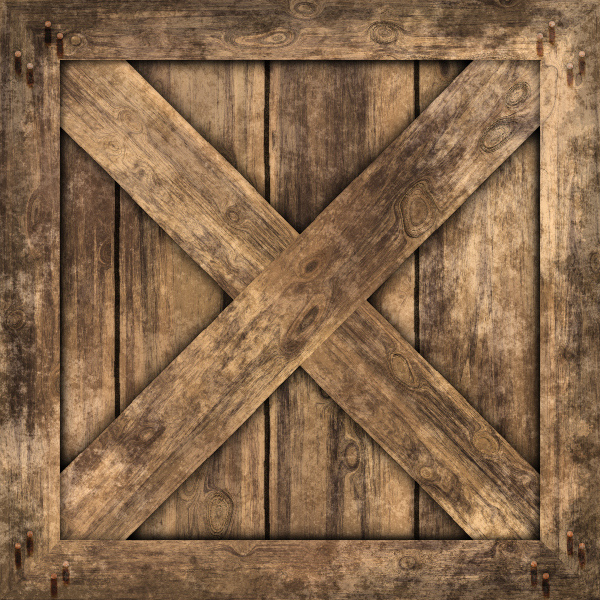# Textures

So far we can render objects like cubes or spheres and make them look nice under lights. But we usually don’t want to render cubes and spheres, but crates and planets, dice and marbles.

Consider a wooden crate. How do you turn a cube into a wooden crate? One option is to add a lot of triangles to replicate the grain of the wood, the heads of the nails, and so on. This will work, but it adds a lot of geometric complexity to the scene, with the performance hit it entails.

Another option is to fake it: take the flat surface of the cube and just paint something that looks like wood on top of it. Unless you’re looking at the crate from up close, you’ll never notice the difference.

We’re going to follow the second approach. The first thing we need is an image to paint on top of the surface; in this context, we call this image a texture, even though it’s exactly the opposite of what we call the texture of an object - whether it’s rough or soft, etc. Here’s a “wood crate” texture:Texture by Filter Forge - Attribution 2.0 Generic (CC BY 2.0)

The next thing we need is to specify how this texture is applied to the model. We can define this mapping on a per-triangle basis, by specifying what points of the texture should go in each vertex of the triangle:

Note that it’s perfectly possible to warp a texture or to use only parts of a texture by playing with the texture coordinates at each vertex.

To define this mapping, we use a coordinate system to specify points in the texture; we call these coordinates $$u$$ and $$v$$, to avoid confusion with $$x$$ and $$y$$ which generally represent pixels in the canvas. We also declare that $$u$$ and $$v$$ are real values in the range $$[0, 1]$$, regardless of the actual pixel dimensions of the image used as a texture. This is very convenient for several reasons; for example, you may want to use a lower- or higher- resolution texture depending on how much RAM you have available, without having to modify the model itself.

The basic idea of texture mapping is simple: compute the $$(u, v)$$ coordinates for each pixel of the triangle, fetch the appropriate texel (that is, texture element) from the texture, and paint the pixel with that color. A given pair $$(u, v)$$ in a texture of dimensions $$(w, h)$$ maps to the texel at $$(u (w-1), v (h-1))$$.

But we only have $$u$$ and $$v$$ coordinates for the three vertexes of the triangle, and we need them for each pixel… and by now you probably see where this is going. Yes, linear interpolation. We use attribute mapping to interpolate the values of $$u$$ and $$v$$ across the face of the triangle, giving us $$(u, v)$$ at each pixel; we paint the pixel with the appropriate color taken from the texture (possibly modified by lighting), and we get…

…underwhelming results. The crates look relatively OK, but if you pay close attention to the diagonal planks, it’s clear they look slightly deformed.

What went wrong?

Again, we fell into the trap of assuming things that weren’t true; namely, that $$u$$ and $$v$$ vary linearly across the screen. This clearly isn’t the case. Consider the wall of a very long corridor painted with alternating vertical black and white stripes. As the wall goes into the distance, we should see the stripes get thinner and thinner. However, if we assume the $$u$$ coordinate varies linearly with $$x'$$, this isn’t the case:

The situation is extremely similar to the one we encountered in the Depth buffering chapter, and the solution is also very similar: although $$u$$ and $$v$$ aren’t linear in screen coordinates, $$u \over z$$ and $$v \over z$$ are1. Since we already have interpolated values of $$1 \over z$$ at each pixel, it’s enough to interpolate $$u \over z$$ and $$v \over z$$ and get $$u$$ and $$v$$ back:

$u = { {u \over z} \over {1 \over z}}$

$v = { {v \over z} \over {1 \over z}}$

This yields the expected results:

Source code and live demo >>

(TO DO)

(TO DO)

## MIP maps

(TO DO)

Found an error? Everything is in Github.

1. The proof is very similar to the $${1 \over z}$$ proof: consider that $$u$$ varies linearly in 3D space, substitute $$X$$ and $$Y$$ with their screen-space expressions.